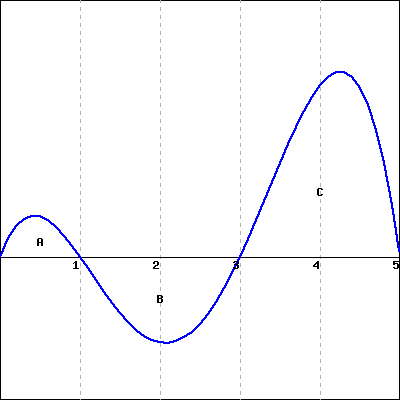Consider the graph of $g(t)$ shown below.(Click on the graph for a larger version.)

If the areas A, B and C are all defined to be the areas between the graph of $g(t)$ and the $x$-axis and are, respectively, 5, 19 and 41, carefully sketch the graph of an antiderivative $G(t)$ of $g(t)$ satisfying $G(0) = 15$. Label each critical point of $G(t)$ with its coordinates.

Then enter the critical points below, as a list of ordered pairs (that is, if you found critical points at $x=2$, $y=3$ and $x=3$, $y=4$, you would enter (2,3), (3,4)).
critical points =